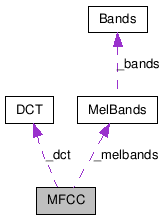# MFCC Class Reference

Algorithm to calculate the Mel-frequency Cepstrum Coefficients of vectors of Real values. More...

`#include <MFCC.h>`

Collaboration diagram for MFCC:[legend]

List of all members.

## Public Member Functions

MFCC (Real lowFrequency=300.0, Real highFrequency=16000.0, int bandCount=40.0, Real samplerate=44100.0, int fftSize=1024, int coefficientCount=13, Real minSpectrum=1e-10, Real power=1.0)
~MFCC ()
void process (const MatrixXR &spectrums, MatrixXR *mfccCoefficients)
int coefficientCount () const
void setCoefficientCount (int count, bool callSetup=true)
int bandCount () const
void setBandCount (int count, bool callSetup=true)
Real lowFrequency () const
void setLowFrequency (Real frequency, bool callSetup=true)
Real highFrequency () const
void setHighFrequency (Real frequency, bool callSetup=true)
Real samplerate () const
void setSamplerate (Real frequency, bool callSetup=true)
int fftSize () const
void setFftSize (int size, bool callSetup=true)
Real power () const
void setPower (Real factor, bool callSetup=true)

## Detailed Description

Algorithm to calculate the Mel-frequency Cepstrum Coefficients of vectors of Real values.

This class represents an object to perform a Mel-frequency Cepstrum Coefficients (MFCC) on vectors of Real values. Which is a useful technique creating a sparse representation of a spectrum magnitude. The algorithm estimates a set of M coefficients which should be similar for perceptually similar sounds.

The MFCCs are calculated by taking the Discrete Cosine Transform (DCT) of the values of the Mel log powers. The Mel log powers are calculated by applying the logarithm to the vaules of the Mel Bands (MelBands). The Mel Bands are triangular overlapping windows applied on the power spectrum mapped onto the Mel-frequency scale.

The samplerate and FFT size of the input spectrum are specified using setSamplerate() and setFftSize().

The frequency limits of the Mel scale mapping are specified using setLowFrequency() and setHighFrequency().

The number of Mel bands is specified using setBandCount().

The number of resulting DCT coefficients is specified by setCoefficientCount().

MelBands, Bands, LPC

## Constructor & Destructor Documentation

 MFCC ( Real lowFrequency = `300.0`, Real highFrequency = `16000.0`, int bandCount = `40.0`, Real samplerate = `44100.0`, int fftSize = `1024`, int coefficientCount = `13`, Real minSpectrum = `1e-10`, Real power = `1.0` )

Constructs an MFCC object with the specified lowFrequency, highFrequency, bandCount, samplerate, fftSize, coefficientCount, minSpectrum and power settings.

Parameters:
 lowFrequency frequency of the lowest Mel band, must be greater than zero 0 and lower than half the samplerate. highFrequency frequency of the highest Mel band, must be greater than zero 0 and lower than half the samplerate. bandCount number of Mel bands. samplerate samplerate frequency of the input signal. fftSize size of the FFT. coefficientCount number of DCT coefficients to be estimated. minSpectrum value to which the spectrum is clipped before performing the logarithm. power value to which to power the band values before performing the DCT.

 ~MFCC ( )

Destroys the algorithm and frees its resources.

## Member Function Documentation

 void process ( const MatrixXR & spectrums, MatrixXR * mfccCoefficients )

Performs an MFCC on each of the rows of frames. Puts the resulting MFCC coefficients in the rows of mfccCoefficients.

Parameters:
 spectrums matrix of Real values representing one spectrum magnitude per row. The number of columns of spectrum must be equal to the fftSize / 2 + 1 where fftSize is specified using setFftSize(). mfccCoefficients pointer to a matrix of Real values for the MFCC coefficients. The matrix should have the same number of rows as spectrums and coefficientCount columns.
Note that if the output matrices are not of the required sizes they will be resized, reallocating a new memory space if necessary.

References DCT::process(), and MelBands::process().

 int coefficientCount ( ) const

Returns the number of coefficients to be calculated. The default is 13.

setCoefficientCount()

 void setCoefficientCount ( int count, bool callSetup = `true` )

Specifies the count of coefficients to be calculated. The given count must be in the range between 0 and (input size - 1).

coefficientCount()

Referenced by MFCC().

 int bandCount ( ) const

Returns the number of bands to be performed. The default is 40.

setBandCount()

 void setBandCount ( int count, bool callSetup = `true` )

Specifies the count of bands to be performed.

bandCount()

Referenced by MFCC().

 Real lowFrequency ( ) const

Return the low frequency of the MFCC. The default is 300.0.

lowFrequency, highFrequency, setLowFrequency, setHighFrequency

 void setLowFrequency ( Real frequency, bool callSetup = `true` )

Specifies the low frequency of the MFCC. The given frequency must be in the range of 0 to the samplerate / 2.

lowFrequency, highFrequency, setHighFrequency

Referenced by MFCC().

 Real highFrequency ( ) const

Return the high frequency of the MFCC. The default is 16000.0.

lowFrequency, setLowFrequency, setHighFrequency

 void setHighFrequency ( Real frequency, bool callSetup = `true` )

Specifies the high frequency of the MFCC. The given frequency must be in the range of 0 to the samplerate / 2.

lowFrequency, highFrequency, setLowFrequency

Referenced by MFCC().

 Real samplerate ( ) const

Return the samplerate frequency of the input signal. The default is 44100.0.

setSamplerate

 void setSamplerate ( Real frequency, bool callSetup = `true` )

Specifies the samplerate frequency of the input signal.

samplerate

Referenced by MFCC().

 int fftSize ( ) const

Returns the size of the FFT that has been performed for the input. The default is 1024.

setFftSize()

 void setFftSize ( int size, bool callSetup = `true` )

Specifies the size of the FFT that has been performed for the input. The given size must be higher than 0.

fftSize()

Referenced by MFCC().

 Real power ( ) const

Return the factor to which the bands are powered. The default is 1.0.

setPower

 void setPower ( Real factor, bool callSetup = `true` )

Specifies the factor to which the bands are powered.

Generated on Tue Mar 31 20:38:33 2009 for Loudia by1.5.6